Porankiewicz, B., Wieczorek, D., Djurkovic, M., Idzikowski, I., and Węgrzyne, Z. (2021). "Modelling cutting forces using the moduli of elasticity in oak peripheral milling," BioResources 16(1), 1424-1437.

#### Abstract

This article presents an attempt to estimate the nonlinear, multivariable dependence between the main (tangential) cutting force (FC) and the processing parameters and moduli of elasticity of oak wood (Quercus robur) during peripheral milling with a straight edge. The analysis indicated that the tangential force (FC) was affected by cutting depth (cD), feed rate per tooth (fZ), rake angle (γF), elastic modulus by stretching along the grain (ESA), elastic modulus by stretching perpendicular to the grain (ESP), elastic modulus by compression along the grain (ECA), and the elastic modulus by compression perpendicular to the grain (ECP). It was found that the elastic moduli (ESA, ESP, ECA, ECP) very well described the mechanical properties of processed wood. Several interactions between the examined parameters (namely, ESA·γF, ESP·γF, ECP·γF, fZ·γF, and fZ·cD) were confirmed in the developed relationship FC = f(ESA, ESP, ECA, ECP, fZ, cD, γF).

Modelling Cutting Forces using the Moduli of Elasticity in Oak Peripheral Milling

Bolesław Porankiewicz,a,* Daria Wieczorek,b Marija Djurkovic,c Ireneusz Idzikowski,a and Zbigniew Węgrzyn d

This article presents an attempt to estimate the nonlinear, multivariable dependence between the main (tangential) cutting force (FC) and the processing parameters and moduli of elasticity of oak wood (Quercus robur) during peripheral milling with a straight edge. The analysis indicated that the tangential force (FC) was affected by cutting depth (cD), feed rate per tooth (fZ), rake angle (γF), elastic modulus by stretching along the grain (ESA), elastic modulus by stretching perpendicular to the grain (ESP), elastic modulus by compression along the grain (ECA), and the elastic modulus by compression perpendicular to the grain (ECP). It was found that the elastic moduli (ESAESPECAECP) very well described the mechanical properties of processed wood. Several interactions between the examined parameters (namely, ESA·γFESP·γFECP·γFfZ·γF, and fZ·cD) were confirmed in the developed relationship FC = f(ESAESPECAECPfZcDγF).

Keywords: Oak wood; Properties of wood; Machining parameters; Peripheral milling; Main cutting force

Contact information: a: Lab-Tech, Radomsko, Poland; b: Poznan University of Economics and Business, Institute of Quality Science, Department of Technology and Instrumental Analysis, al. Niepodległości 10, 61-875 Poznań, Poland; c: Department of Wood Science and Technology, University of Belgrade Faculty of Forestry, Belgrade, Serbia; d: University of Zielona Góra, Faculty of Mechanical Engineering, Institute of Mechanical Engineering, Zielona Góra, Poland; *Corresponding author: poranek@amu.edu.pl

INTRODUCTION

Throughout the years, various studies have examined the cutting process, considering wood properties and cutting conditions (Kivimaa 1950; Koch 1964; Woodson and Koch 1970; Axelsson et al. 1993), as well as grain orientation and wood structure (Cyra and Tanaka 1997; Goli et al. 2003; Porankiewicz and Goli 2014; Curti et al. 2019), to predict the results of machining. The calculation of the optimum values of the machining parameters could serve as the basis for predicting the behavior of the material in the machining process, the outcome of machining, and, above all, the quality of the machined surface. In general, cutting forces represent the basic parameters of the cutting process mechanics that demonstrate the basic characteristics of the cutting process conditions and behavior. The main reason for this is that the measurement of cutting forces is a powerful tool, allowing the building of physico-mechanical cutting models for a better understanding of the phenomena observed during cutting. The knowledge of the functions of cutting forces serves as the basis for ensuring a rational and economic use of means of production under the given conditions. In addition, optimization of the machining process is ensured.

There are various models in the literature that deal with the problem of calculating cutting forces. One of them is the method of coefficients (Afanasev 1961; Beršadskij 1967; Amalitskij and Lûbčenko 1977; Orlicz 1982; Goglia 1994). The aforementioned authors start from the so-called reference unit cutting resistance measured under accurately defined conditions. Specific cutting resistances for certain materials and processing conditions are expressed as products of reference unit cutting resistances and appropriate coefficients of correction, whose values ​​are given in adequate tables. Every change in one of the influencing parameters will also change the value of the unit cutting resistance. The principal cutting force is obtained by multiplying the calculated coefficient by the cross-sectional area of the shavings for the corresponding type of processing. The differences between the values of the forces obtained by the method of coefficients and the values of the forces measured experimentally are considerable (Mandić et al. 2014; Đurković and Danon 2017). One reason for such large differences is the absence of the physical and mechanical properties of the wood in the mentioned models, as they are commonly represented only by the correction coefficient for the wood species. Another reason is that the correction coefficients are not the result of a multifactorial experiment, so interdependencies between individual factors are not included in the model. There are several other possible reasons, and the conclusion would be that, to control the cutting process to achieve a sufficiently good estimate of the levels and characters of the cutting forces, the influences of the anatomical, physical, and mechanical properties of the wood should be included in the model. However, these models are usually based on extensive experiments, which are most often performed on specially designed laboratory equipment and at low cutting speeds. Therefore, they do not provide a sufficient level of generality.

There are models in the literature that include wood properties (Axelsson et al. 1993; Porankiewicz et al. 2011; Naylor et al. 2012; Mandić et al. 2015). Axelsson et al. (1993) gave a polynomial model equation with interactions based on multifactor experiments performed on specifically designed laboratory machines. The model includes the impacts of material properties, cutting conditions, and the angle parameter of the blades on the tangential force (FC). The model published by Porankiewicz et al. (2011) was created and verified based on the experimental results of Axelsson et al. (1993). The model gives statistical equations for the tangential (FC) and normal (FN) cutting forces as functions of the physical properties of the samples, tool characteristics, and characteristics of the processing regime. Naylor et al. (2012) formed a model for the prediction of cutting forces and included the mechanical properties of the wood in the variables, in addition to wood density and moisture. The model published by Mandić et al. (2015) gives statistical equations for the main (tangential) force (FC) as a function of the density (D), moisture content (mC), Brinell hardness (H), bending strength (RB), the modulus of elasticity (E), feed rate per tooth (fZ), rake angle (γF), and cutting depth (cD).

Đurković and Danon (2017) give a comparative analysis of calculated values (according to the method of coefficients and the model of Axelsson et al. (1993)) and measured values of cutting forces. The final conclusion is that the given models are simple to use and suitable for application if comparison is done between the impacts of some factors on the mechanics of cutting, but they are not suitable for quantification of their impacts, i.e., calculations of specific values of the cutting forces. A major obstacle to comparing the available models is the incompatibility of the cutting parameters’ ranges of variation and the values of fixed parameters for which the experiments were performed.

This study attempted to estimate the relationship the main (tangential) cutting force (FC) and machining parameters (cutting depth (cD), feed rate per edge (fZ), and rake angle (γF)) during peripheral milling of oak wood, as well as some mechanical properties (modulus of elasticity by stretching along the wood grain (ESA), modulus of elasticity by stretching perpendicularly to the grain (ESP), modulus of elasticity by compression along the wood grain (ECA), and modulus of elasticity by compression perpendicularly to the grain (ECP)). The other parameters, including cutting edge round up radius (ρ), cutting speed (vC), diameter of the cutter (DC), cutter width (WC), and the number of cutting edges (z), were kept constant.

EXPERIMENTAL

The minimum and maximum values of the ESAESPECAECPand DEN are of <1164.54 MPa; 1419.26 MPa>, <54.18 MPa; 74.31MPa>, <782.1 MPa; 866.6 MPa> and <455.7 MPa; 519.8 MPa>; <692 kg/m3; 785 kg/m3> respectively.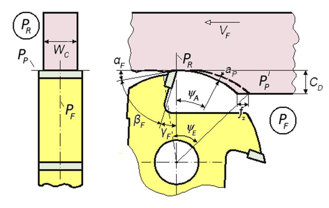Fig. 1. Cutting situation in longitudinal milling using the cutter with a hole: aP – average thickness of cutting layer; ψA – average cutter-workpiece engagement angle; ψE – cutter-workpiece engagement angle; PF – working plane; PR – main plane; PP – back plane

Experimental research was performed using a table milling machine on a Minimax CU 410K combined machine (SCM, Rimini, Italy) equipped with a 3 kW three-phase asynchronous electrical motor (voltage 220/380 V, frequency 50 Hz, power factor 0.83) at the University of Belgrade, Faculty of Forestry Center for Machines and Apparatuses. Accessory motion was achieved using an external, removable feed device (Maggi Engineering Vario feeder, Maggi Technology, Certado, Italy), with speeds ranging between 3 m/min and 24 m/min, equipped with a 0.45 kW three-phase asynchronous electrical motor.

Testing was performed during opened, up-milling, and peripheral milling processes. The tool used consisted of three milling cutters manufactured by Freud (Straight edge cutter, Udine, Italy). The cutters were equipped with four soldered plates, made of a hard metal cemented carbide (H302). The tools were characterized by the following parameters: diameter (DC = 125 mm), width (WC = 40 mm (Fig.1)), radius of the round up (ρ = 2 μm), roughness of rake surfaces (R = 0.15 μm), and roughness of clearance surfaces (R = 0.18 μm). The average wood grain orientation angle toward the cutting speed velocity vector (φV) was in the range of 0.1241 rad to 0.1908 rad, and that toward the cutting plane (φS) was in the range of 0.1241 rad to 0.1908 rad. The orientation angle of the wood grain towards the cutting edge (φK) was 90°. Testing was performed with a constant number of rotations per minute (RPM) of the working spindle (5860 RPM, i.e., at a constant cutting speed (vC) of 38.35 m/s). Values of the other processing parameters are shown in Table 1.

Table 1. Peripheral Milling Process Parameters of Oak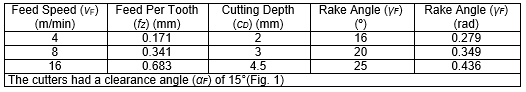The cutting power was measured indirectly, through the power input of the propulsion electric motor at each passage of the tool during the peripheral milling of the oak samples. A measuring-acquisition device (SRD1, Unolux, Belgrade, Serbia) with a sampling frequency of 100 Hz was used for data measurement, acquisition, analysis, and processing. It allowed data to be stored and displayed later (Mandić et al. 2015).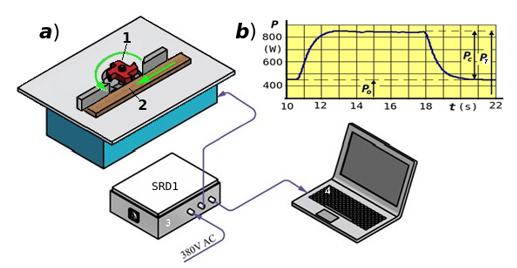Fig. 2. (a) Experimental setup; (b) typical record of cutting power measurements during peripheral milling

Figure 2a shows the installation for cutting power recording with the following elements: (1) tool, (2) oak test sample for milling power measurement, (3) SRD1 measuring and acquisition device, and (4) a computer configured to work with the data acquisition system. Figure 2b presents a typical record of cutting power and the manner of its processing. It is clear from the graph that the power required for cutting (PC) is the difference between the values of total power (PT) and power when idle (PO) (Eq. 1).

PC = PT – PO (W) (1)

Mean values of the main cutting force (FA) depended on average values of the measured cutting powers (PCA) (W), based on a higher number of cuts on the same test sample, and cutting speed (vC) (m/s) (Eq. 2):

FA = PCA / vC (N) (2)

The calculated value of the mean force (FA) represents the mean force for one rotation of the tool, so it includes idle time between the blades (Fig. 3).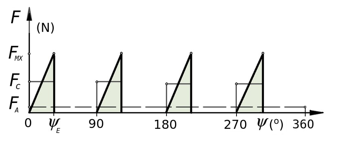Fig. 3. Change of the main cutting force for one cutter revolution: F – tangential (main) cutting force during one revolution of the cutter; Ψ – cutting edge rotation angle; ΨE – cutting edge-workpiece engagement angle

To obtain the mean main cutting force per cutter edge (FC), it is necessary to correct the value of the mean force (FA) (Eq. 3),

FC = 2 · π · FA / (n · ψE) (3)

where FC is the mean cutting force per blade edge (N), n is the number of milling cutter blades, and ψE is the cutter-workpiece engagement angle (rad) (Fig. 1).

The cutter-workpiece engagement angle can be calculated as in Eq. 4,

ψE = arccos((R – cD) / R) + arcsin(fZ / (2 · R)) (4)

where R is the milling cutter radius (mm), cD is the cutting depth (mm), and fZ is the feed rate per tooth (mm) (Fig. 1).

For each of the 22 specimens, moduli of elasticity were evaluated for a moisture content of 7%, after keeping the wood specimens in an air conditioning chamber (Wamed KBK-100, Warsaw, Poland) for 3 months. The dimensions of the cuboid wood specimens were measured using digital calipers (Kraft & Dele KD10298, Starogard Gdański, Poland): for stretching along the wood grain, 140 mm × 20 mm × 4 mm, stretching along the dimension of 140 mm; for stretching perpendicular to the wood grain, 50 mm × 10 mm × 10 mm, stretching along the dimension of 50 mm; and for compression, 20 mm × 20 mm × 30 mm, compressing along the dimension of 30 mm, along and perpendicular to the wood grain. Due to wood limitations, the specimens were not shaped exactly according to standards.

The total number of measuring points in the experimental matrix was 196. As already mentioned, the testing was conducted for various combinations of cutting process parameters. The number of repetitions for each combination of machining parameters is given in Table 2.

Table 2. Number of Repetitions for Each Combination of Machining Parameters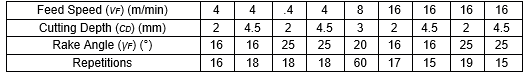The derived values of the mean chip thickness of the cutting layer (aP) and the angle between the cutting velocity vector (vC) and the wood grain (φV) are given in Table 3.

Table 3. Derived Peripheral Milling Process Parameters of Oak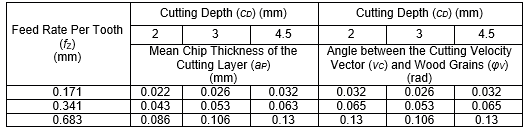Based on the calculated average forces, the relationship FC = f(ESAESPECAECPfZγFcD) was estimated in preliminary calculations for linear, polynomial, and power functions, with and without interactions. The formula should fit the experimental matrix with the lowest sum of squared residuals (SK). This will provide the lowest standard deviation of residuals (SR) and the highest correlation coefficient between predicted and observed values (R). The use of less complicated models will result in decreased approximation quality, with larger values of SK and SR and a lower R. The statistical formula determined cannot be valid outside of the ranges of independent variables chosen within the experimental matrix. With incomplete experimental matrices and complicated statistical formulas with interactions, all predicted values of the dependent variable can have greater error. Moreover, years of experience suggests that using a simple formula to fit an experimental matrix can reverse the influences of any independent variables with small importance. Only more complex mathematical formulas will ensure the correct influence of the less important independent variables. A non-linear multivariable formula with interactions (Eqs. 5 to 8) appeared to be the most appropriate in the present analysis,

FCP = a9 · eA + B + C (5)

where FCP is the predicted tangential cutting force (N), and

A = a2 · ESA + a3 · ESP a4 · ECA + a5 · ECP + a6 · fZ + a7 · γF + a8 · cD (6)

B = a10 · ESA · γFa16 + a11 · ESP · γFa17 + a12 · ECA · γFa18 + a13 · ECP · γFa19 (7)

C = a14 · fZ · γFa20 + a15 · fZ · cDa21 + a1 (8)

The estimators (a1 to a21) were obtained from the 196 data points of the incomplete experimental matrix. The coefficient of relative importance (CRI) (Eq. 9) was used for elimination of unimportant or low-importance estimators during the evaluation of the chosen statistical formula,

CRI = ((SK + SK0i) / SK) · 100 (%) (9)

where SK0i is the sum of squared residuals for the estimator ai = 0, where ai is the estimator with the number i in the statistical formula evaluated.

A flow chart of the optimization program is shown in Fig. 4. For the characterization of the approximation quality, the sum of squared residuals (SK), the standard deviation of residuals (SR), and the correlation coefficient between the predicted and observed values (R), as well as R2, were applied. The calculation was performed using an optimization program based on a least squares method, combined with gradient and Monte Carlo methods (Fig. 4), at the Poznań Networking & Supercomputing Centre (PCSS) on an SGI Altix 3700 machine (Mountain View, CA, USA).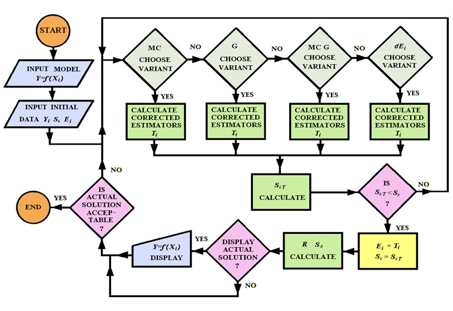Fig. 4. Flow chart of the optimization program; variants: MC – Monte Carlo, G – gradient, MC G – combined MC and G, SK – sum of squared residuals, R – correlation coefficient, SR – standard deviation of residuals

RESULTS AND DISCUSSION

The final shape of the approximated dependence (Eqs. 5 to 8) was determined using the optimization program shown in Fig. 4. The following estimators were evaluated: a1 = 17.94648; a2 = 0.025359; a3 = -0.10558; a4 = -0.030909; a5 = 0.012781; a6 =3.61247; a7 = -0.17306; a8 = 0; a9 = 0.0053921; a10 = -1.3162 · 10 -17 ; a11 = 0; a12 = 0.11665; a13 = -24.94816; a14 = 7.8172 · 10 -17; a15 = 1.0392 · 10 -13 ; a16 = 11.070646; a17 = 0; a18 = 0; a19 = -1.81757; a20 = 12.67553; a21 = 22.40637.

It was decided to round the estimator values to the 5 decimal (or significant) place, which produced an acceptable deterioration of the fit of less than 0.02%. Decreasing the number of rounded decimal (or significant)) digits to 4, 3 and 2 caused deteriorations of the fit of as much as 3.3%, 27.9% and 1616.8%, respectively.

The coefficients of relative importance (CRI) for the estimators had the following values: CRI1 = 5668.3; CRI2 = 8198.7; CRI3 = 1.37 · 1010; CRI4 = 8.97 · 1013; CRI5 = 8170.5; CRI6 = 6785.9; CRI7 = 3.69 · 106; CRI8 = 0; CRI9 = 8198.7; CRI10 = 13466.5; CRI11 = 0; CRI12 = 1.65 · 105; CRI13 = 67744.6; CRI14 = 2475.7; CRI15 = 2683.4; CRI16 = 13466.5; CRI17 = 0; CRI18 = 0; CRI19 = 2.56 · 109; CRI20 = 2475.7; CRI21 = 2683.4.

For each of the 25 combinations of input data, the predicted tangential cutting force (FCP) was calculated using Eqs. 5 to 8. The results, together with the observed values of the main cutting force (FCO), are shown in Fig. 5. The approximation quality of the fit can be characterized by these quantifiers: SK = 1113.76, R = 0.994, R2 = 0.987, and SR = 2.39 N. After replacing the estimators (a1 to a21) by their numerical values, Eqs. 5 to 8 take the following forms:

FCP = 0.005392 · eA + B + C (10)

where:

A = 0.025359 · ESA – 0.10568 · ESP – 0.030909 · ECA + 0.012781 · ECP + 3.61247 · fZ – 0.17306 · γF (11)

B = -1.3162 · 10-17 · ESA · γF11.070646 + 0.11665 · ECA – 24.94816 · ECP · γF-1.81757 –17 (12)

C = 7.8172 · 10-17 · fZ · γF12.67553 + 1.0392 · 10-13 · fZ · cD22.40646 + 17.94648 (13)

Figure 5 shows that the maximum deviation from Eqs. 10 to 13 was as high as S= 6.9 N.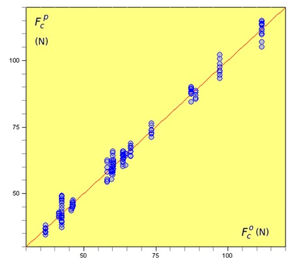Fig. 5. Plot of the observed main force (FCO) and the predicted values (FCP), according to Eqs. 10 to 13

These equations provide a strong link between the observed cutting force (FCO) and the predicted cutting force (FCP) and can be used to analyze the influence of specific inputs on the predicted cutting forces. Equations 5 to 8 show the following interactions: ESA·γFECP·γFfZ·γF, and fZ·cD. In the range of values of the independent variables from minimum to maximum, these interactions were changing the value of FCP by more than 40%. The interaction ECA·γF0 was eliminated from Eqs. 5 to 8 during the calculation process.

Figure 6 shows the relationship of the predicted main cutting force (FCP) to the feed rate per tooth (fZ) and the rake angle (γF). As shown, FCP, for the largest fZ, strongly, in a parabolic increasing manner, depended on fZ. An increase in fZ increased FCP more at lower values of γF. The average change of FCP with an increase of the feed rate per tooth (fZ) by 0.1 mm was between 8 N and 12 N, depending on the value of the rake angle (γF). An increase in γF decreased FCP for the largest feed rate per tooth (fZ). As fZ decreased to less than approximately 0.6 mm, a maximum started to appear. For the lowest fZ, the influence of γF on FCP was smaller, with a maximum at a γF of approximately 21°. A decrease in FCP = f(γF) for values of γF greater than approximately 21° is not reported in the literature.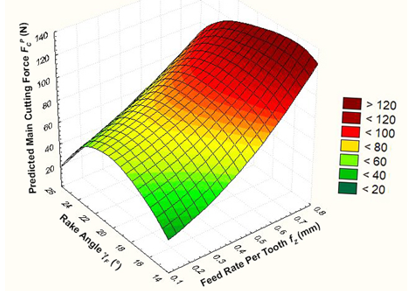Fig. 6. Plot of the relationships among the predicted main cutting force (FCP) (N), γF (o), and fZ (mm), according to Eqs. 10 to 13; ESA = 1419.3 MPa; ESP = 78.11 MPa; ECA = 882.1 MPa; ECP = 455.7 MPa; cD = 4.5 mm

Figure 7 shows the relationship of the predicted main force (FCP) to the moduli of elasticity (ESA and ESP). As shown, with an increase in ESA in the range from approximately 1342 MPa to 1419.26 MPa, for a minimum ESP = 54.15 MPa, the tangential cutting force (FCP) increased fast in a nonlinear, parabolic manner. For a maximum ESP = 78.1 MPa, the tangential cutting force (FCP) increased much more slowly in a nonlinear, parabolic manner. The influence of the modulus of elasticity (ESP) on the tangential cutting force (FCP) was opposite. When ESA ranged from 1164 MPa to approximately 1266 MPa, FCP had little dependence on either ESA or ESP at any point in the range of variation of ESP.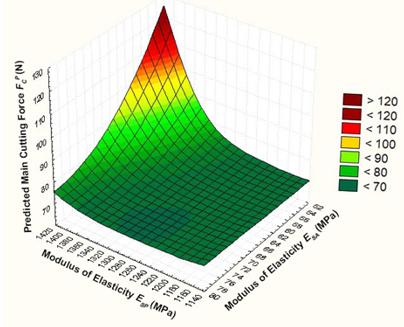Fig. 7. Plot of the relationships among the predicted main cutting force (FCP) (N), ESA (MPa), and ESP (MPa), according to Eqs. 10 to 13; ECA = 866.6 MPa; ECP = 519.8 MPa; γF = 17°; fZ = 0.68 mm; cD = 4.5 mm